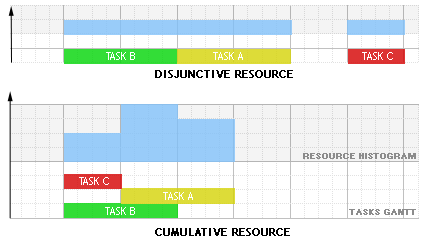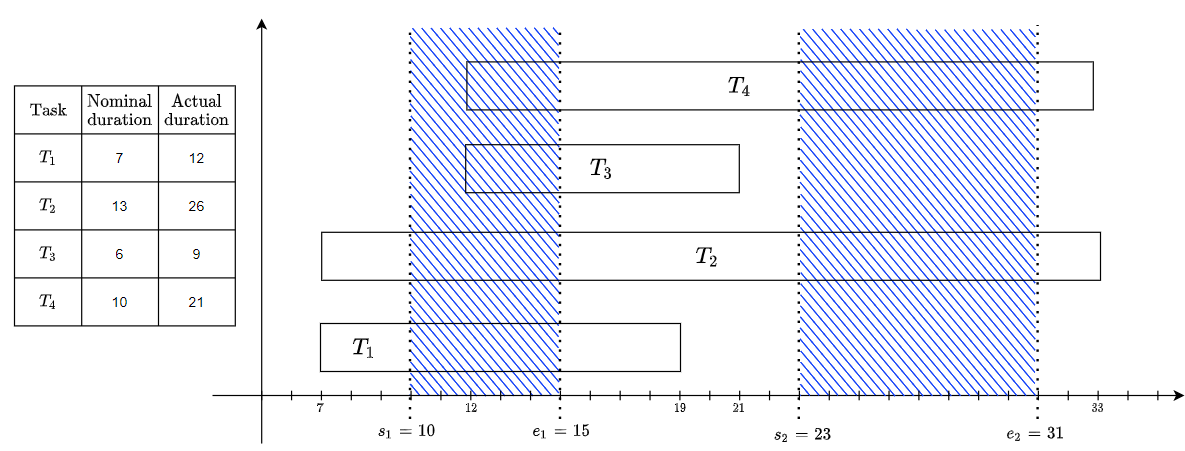# KResource¶

class KResource : public KPtrArray<KResource>

Resources (machines, raw material etc) can be of two different types :

• Disjunctive when the resource can process only one task at a time (represented by the class KUnaryResource).

• Cumulative when the resource can process several tasks at the same time (represented by the class KDiscreteResource).

Traditional examples of disjunctive resources are Jobshop problems, cumulative resources are heavily used for the Resource-Constrained Project Scheduling Problem (RCPSP). Note that a disjunctive resource is semantically equivalent to a cumulative resource with maximal capacity one and unit resource usage for each task using this resource but this equivalence does not hold in terms of constraint propagation.

The following schema shows an example with three tasks A,B and C executing on a disjunctive resource and on a cumulative resource with resource usage 3 for task A, 1 for task B and 1 for task C :Tasks may require, provide, consume and produce resources :

• A task requires a resource if some amount of the resource capacity must be made available for the execution of the activity. The capacity is renewable which means that the required capacity is available after the end of the task.

• A task provides a resource if some amount of the resource capacity is made available through the execution of the task. The capacity is renewable which means that the provided capacity is available only during the execution of the task.

• A task consumes a resource if some amount of the resource capacity must be made available for the execution of the task and the capacity is non-renewable which means that the consumed capacity if no longer available at the end of the task.

• A task produces a resource if some amount of the resource capacity is made available through the execution of the task and the capacity is non-renewable which means that the produced capacity is definitively available after the starting of the task.

Subclassed by KDiscreteResource, KUnaryResource

Public Functions

KResource()

Empty constructor

KResource(KSchedule &schedule)

Basic constructor

KResource(const KResource &toCopy)

Copy constructor

virtual ~KResource()

Destructor

virtual KResource *getCopyPtr() const

Return a copy of this object

virtual void print() const

Pretty printing of this resource.

virtual void print(void *ctx, PrintFunctionPtr *pfp) const

Pretty printing of this resource with a print function pointer.

virtual void close()

Close this resource.

int getIndex() const

Return the unique index of the resource.

const char *getName() const

Return the name of this resource.

void setName(const char *name)

Set the name of this resource.

virtual void printResourceGantt(KSolution &s, int factor) const

Pretty printing the resource Gantt chart in the console

Parameters
• s – Given scheduling solution

• factor – distortion factor to print the Gantt in the console

virtual void printResourceGantt() const

Pretty printing the resource Gantt chart.

virtual void printTaskGantt(KSolution &s, int factor) const

Pretty printing the task Gantt chart in the console

Parameters
• s – Given scheduling solution

• factor – distortion factor to print the Gantt in the console

Pretty printing the task Gantt chart.

KIntVar *getSlackVar()

Return the KIntVar representing the slack for this resource.

KIntVar *getLCTVar()

Return the KIntVar representing the latest completion time of all the tasks executing on this resource.

KIntVar *getESTVar()

Return the KIntVar representing the earliest starting time of all the tasks executing on this resource.

KIntVar *getDURVar()

Return the KIntVar representing the difference between LST and EST variables.

Return the number of tasks using this resource.

Return task with index ‘index’ in this resource.

virtual bool getIsInstantiated()

Return true if all the tasks on this resource are fixed.

virtual int getInitialCapacity()

Return the capacity at timestep 0.

virtual int getInitialCapacityAt(int t)

Return the initial resource stock at time step `t`

virtual void setInitialCapacityBetween(int t0, int t1, int capa)

Set the initial resource stock between time steps `t0` and `t1` to `capa`

Parameters
• t0 – start of the interval

• t1 – end of the interval

• capa – initial resource stock

virtual int getMaxAvailability()

Return the initial resource storage at time step 0.

virtual int getMaxAvailabilityAt(int t)

Return the initial resource storage at time step t.

virtual void setMaxAvailabilityBetween(int t0, int t1, int capa)

Set the initial resource stock between time steps `t0` and `t1` to `capa`

Parameters
• t0 – start of the interval

• t1 – end of the interval

• capa – initial resource stock

virtual int getMinUsageAt(int t)

Return the initial resource stock at time step t.

virtual void setMinUsageBetween(int t0, int t1, int capa)

Set the initial resource stock between time steps `t0` and `t1` to `capa`

Parameters
• t0 – start of the interval

• t1 – end of the interval

• capa – initial resource storage

Add idle time steps to this resource.

During “idle time steps”, the resource does nothing, i.e. its usage (consumption, production, …) for any task T is set to zero and delayed one time step after (if T is executed on this very time step).

bool isIdleTimeStep(int timestep)

Return true IFF `timestep` is an idle timestep for this resource.

Add a coupled setup time between two tasks for the current resource. This means that if the two given tasks are assigned to the resource, then the start of the second task must be after the end of the first task plus the given duration :

r.assign(t1) and r.assign(t2) => t1.end + d <= t2.start

Set the duration of the task if the task is assigned to this resource.

If the task is assigned to this resource, the duration variable will take the given `duration` value:

`(task.assign(r) = 1) => task.duration = duration`

Note that this is equivalent than setting start based duration with one possible value.

See
Since

13.2.1

Parameters

• duration – The actual duration taken if the task is allocated to the resource

Set a duration computed from the value taken by the start variable if the task is assigned to this resource.

If the task is assigned to this resource, the duration variable will take the given `duration` value if the start of the task is between the given interval:

`(t1 <= task.start <= t2) and (task.assign(r) = 1) => task.duration = duration`

This method can be called successively to specify different durations on different intervals. Calling successively with intersectings intervals will override existing values on the intersection. Also, propagation should not be called between sucessive calls. Note: All values not included in any of the given intervals will be forbidden for the start variable.

See
Since

13.2.1

Parameters

• t1 – The first interval extremity

• t2 – The second interval extremity

• duration – The actual duration taken if the start is in the given interval

When declaring a task having a start based duration (through `setStartBasedDuration` or `setDurationWithIdleTimes`), this method will return the actual duration of task if it begins at `start` timestep.

If the `start` value is not available in the start-based duration domain, `-1` will be returned.

See
Since

13.2.1

void setDurationWithIdleTimes(KTask *task, int duration, const KIntArray &idleStarts, const KIntArray &idleEnds, bool allowStartInIdle)

Set a duration constraint conditional to some idle time windows and on the task assignment.

If the task is assigned to this resource, the then the following statements will be enforced.

From the given nominal `duration`, the duration variable will be constrained to take the following values:

• `duration` if the task does not intersect any idle time window

• `duration` increased by total length of the intersecting idle time windows

For example, if a resource is idle on two time windows [s1, e1) and [s2, e2), then this method declares that the tasks intersecting those idle times windows will be extended.

• If a task T1 starts before s1 but its `duration` make it intersect [s1, e1), then its duration variable will be `T1.duration = duration + e1 - s1`

• If a task T2 starts before s1 but its updated duration make it intersect [s1, e1) and [s2, e2), then its duration variable will be `T2.duration = duration + (e1 - s1) + (e2 - s2)`

• If a task T3 starts in the idle interval [s1, e1), then its duration variable will be `T3.duration = duration + (e1 - T3.start)`

• If a task T4 starts in the idle interval [s1, e1) and its updated duration make it intersect [s2, e2) then its duration variable will be `T4.duration = duration + (e1 - T4.start) + (e2 - s2)`

Note that if `allowStartInIdle` is false then `T3` and `T4` cases will be forbidden. The idle time windows can be given in any order and can be intersecting (union is made). Note that idle time windows are a strict end interval (end time step is not in the interval). If the nominal duration is dependent on the start time of the task, the `updateDurationWithIdleTimes` method can be used instead.See
Since

13.2.1

Parameters

• duration – The expected nominal duration of the task without idle times

• idleStarts – The idle time windows start (must be of same length as `idleEnds`)

• idleEnds – The idle time windows end (must be of same length as `idleStarts`)

• allowStartInIdle – If true, the `task` will be able to start in an idle time window.

Update the current start-based duration constraint with a new idle window.

When having previously set a start-based duration (through `setDuration` or `setStartBasedDuration`), this method will update the start-based durations to simulate idle times windows.

Warning: when adding several idle time windows, they must be added in increasing time order and time windows must be disjoints. If not done this way, durations might be inconsistent. Also, propagation should not be called between sucessive calls.```resource.setDuration(task, 13);
// Adding a first idle time window
int d = resource.getStartBasedDuration(task, 7); // d = 18
// Adding the second idle time window
d = resource.getStartBasedDuration(task, 7); // d = 26
```

See
Since

13.2.1

Parameters
• allowStartInIdle – If true, the `task` will be able to start in the idle time window.